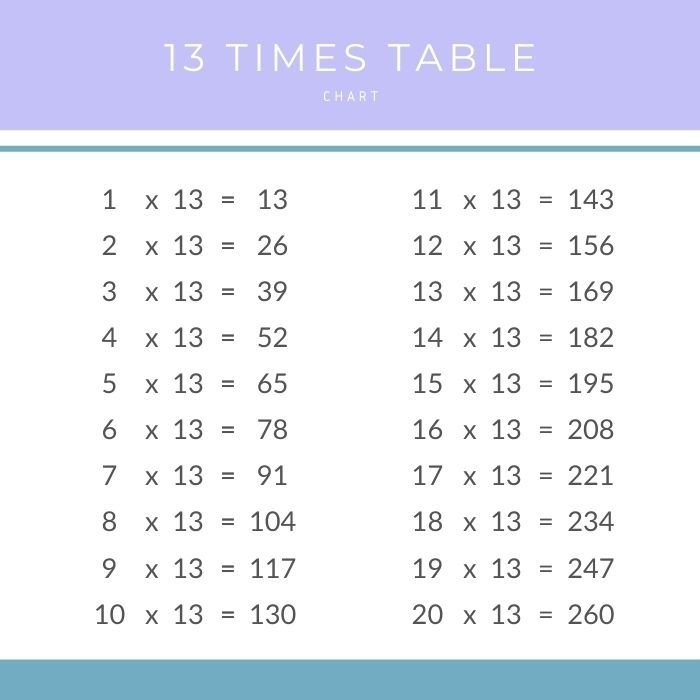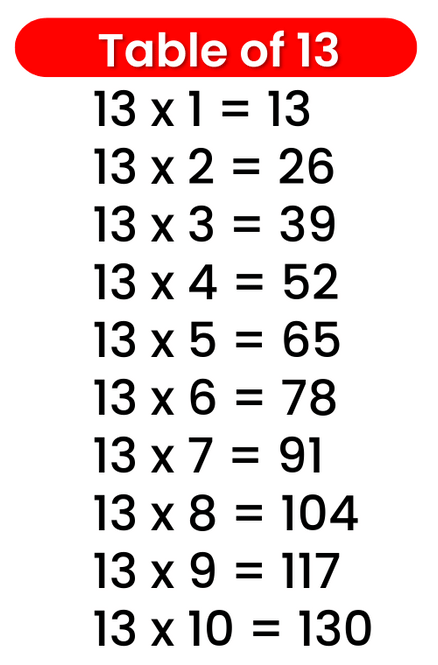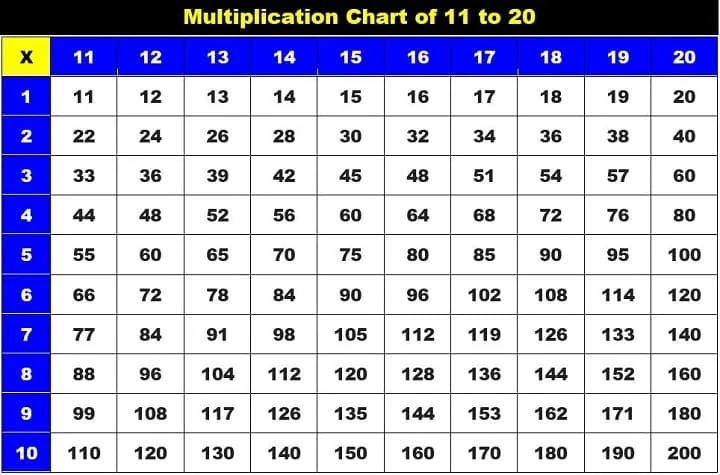# Times Table of 13, 13 ka table

## Times Table of 13, 13 ka table

Maths Multiplication Table of 13, Times Table of 13, 13 ka table, 13 ki Table Chart

13 ×‌ 1 = 13
13 ×‌ 2 = 26
13 ×‌ 3 = 39
13 ×‌ 4 = 52
13 ×‌ 5 = 65
13 ×‌ 6 = 78
13 ×‌ 7 = 91
13 ×‌ 8 = 104
13 ×‌ 9 = 117
13 ×‌ 10 = 130

## 13 ka table### Multiplication Tables of twelve upto 20### Times Table of 13 chart